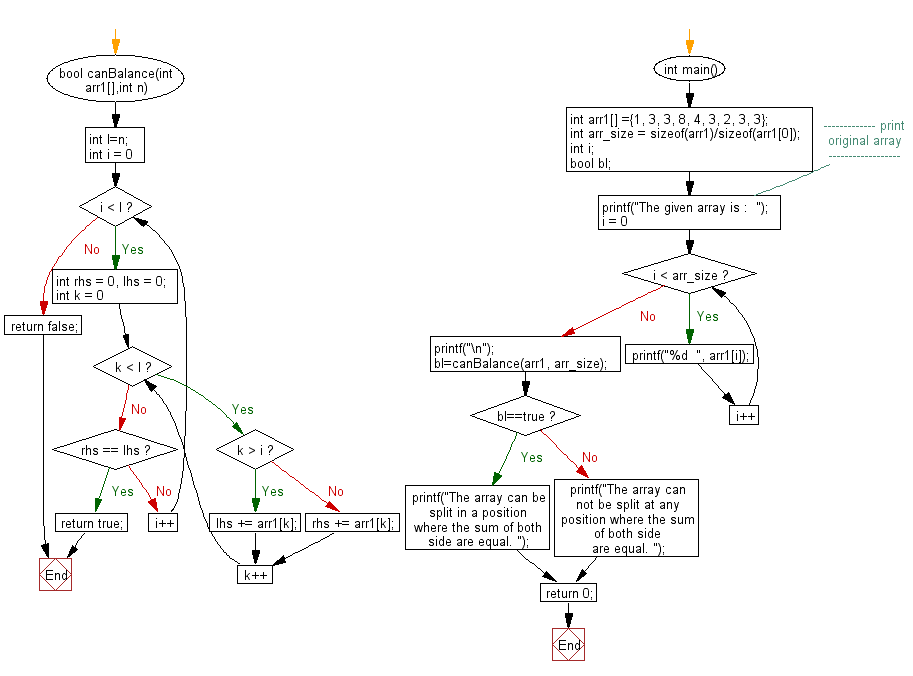﻿ C exercises: Check if an array can be splitted in such a position that, the sum of left side of the splitting is equal to the sum of the right side - w3resource# C Exercises: Check if an array can be splitted in such a position that, the sum of left side of the splitting is equal to the sum of the right side

## C Array: Exercise-99 with Solution

Write a program in C to check if an array can be splitted in such a position that, the sum of left side of the splitting is equal to the sum of the right side.

Sample Solution:

C Code:

``````#include<stdio.h>
# include <stdbool.h>

bool canBalance(int arr1[],int n)
{
int l=n;
for(int i = 0; i < l; i++)
{
int rhs = 0, lhs = 0;
for(int k = 0; k < l; k++)
{
if(k > i)
{
lhs += arr1[k];
}
else
{
rhs += arr1[k];
}
}

if(rhs == lhs)
{
return true;
}
}
return false;
}

int main()
{
int arr1[] ={1, 3, 3, 8, 4, 3, 2, 3, 3};
int arr_size = sizeof(arr1)/sizeof(arr1);
int i;
bool bl;
//------------- print original array ------------------
printf("The given array is :  ");
for(i = 0; i < arr_size; i++)
{
printf("%d  ", arr1[i]);
}
printf("\n");
//------------------------------------------------------
bl=canBalance(arr1, arr_size);
if (bl==true)
printf("The array can be split in a position where the sum of both side are equal. ");
else
printf("The array can not be split at any position where the sum of both side are equal. ");

return 0;
}
```
```

Sample Output:

```The given array is :  1  3  3  8  4  3  2  3  3
The array can be split in a position where the sum of both side are equal.
```

Pictorial Presentation:Flowchart:C Programming Code Editor:

Improve this sample solution and post your code through Disqus.

﻿